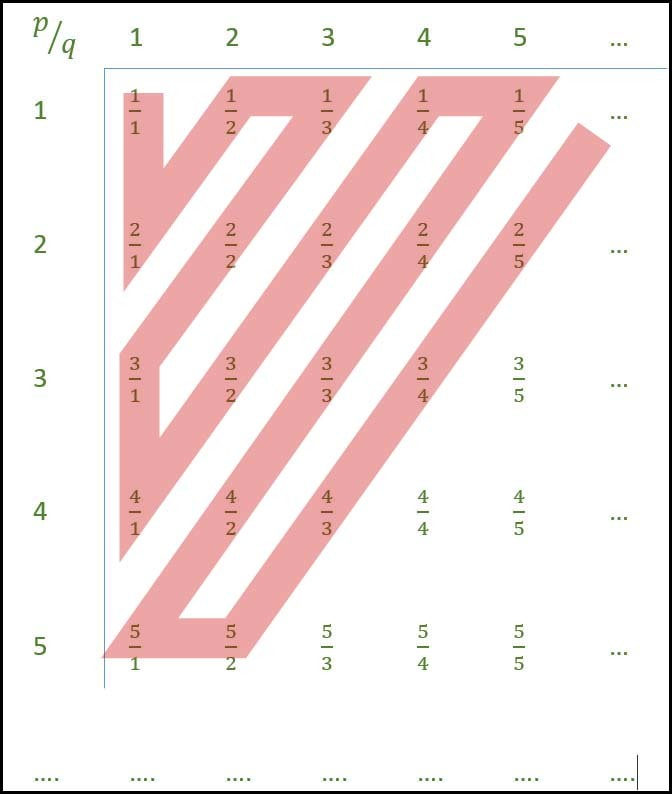# Determining Countability in TOC

Countable Set is a set having cardinality same as that of some subset of N the set of natural numbers . A countable set is the one which is listable.

Cardinality of a countable set can be a finite number. For example, B: {1, 5, 4}, |B| = 3, in this case its termed countably finite or the cardinality of countable set can be infinite. For example, A: {2, 4, 6, 8 …}, in this case its termed countably infinite.

Common Traces for Countable Set:

• Cardinality expressed in formwhere,; m may or may not be ∞
• It has finite elements* only in case of countably finite sets.
• It listable in terms of roaster form* an exhaustive list exists which can include every element atleast once, in case of countably infinite list first few elements followed by three dot ellipsis(…).

Set of Rational numbers is Countably Infinite:Follow along the red line to build roaster set containing all rational numbers. Hence an exhaustive set containing every element atleast once can be build therefore set of rational numbers is countably infinite.

Uncountable Sets:
A set such that its elements cannot be listed, or to put intuitively, there exists no sequence which can list every element of the set atleast once.

Example:

R : {set of real numbers is uncountable}
B : {set of all binary sequences of infinite length} 

Common Traces for Uncountable Set:

• Cardinality expressed in form;
• It is power set of set with infinite elements
• It is equal set to R set of real numbers
• It is equal set to Q set of irrational numbers
• It is non-listable set

Union Operations quick Reference:

A BCountable Countable Countable
Uncountable Uncountable Uncountable
Countable Uncountable Uncountable

Example-1:
Let N be the set of natural numbers. Consider the following sets,

P: Set of Rational numbers (positive and negative)
Q: Set of functions from {0, 1} to N
R: Set of functions from N to {0, 1}
S: Set of finite subsets of N 

Which of the above sets are countable ?
(A) Q and S only
(B) P and S only
(C) P and R only
(D) P, Q and S only

Explanation:
Please see GATE CS 2018 | Question 58

Example-2:
Consider the following sets:

S1: Set of all recursively enumerable languages over the alphabet {0, 1}.
S2: Set of all syntactically valid C programs.
S3: Set of all languages over the alphabet {0, 1}.
S4: Set of all non-regular languages over the alphabet {0, 1}. 

Which of the above sets are uncountable?
(A) S1 and S2
(B) S3 and S4
(C) S1 and S4
(D) S2 and S3

Explanation:
Please see GATE CS 2019 | Question 43

Attention reader! Don’t stop learning now. Get hold of all the important CS Theory concepts for SDE interviews with the CS Theory Course at a student-friendly price and become industry ready.

My Personal Notes arrow_drop_upCheck out this Author's contributed articles.

If you like GeeksforGeeks and would like to contribute, you can also write an article using contribute.geeksforgeeks.org or mail your article to contribute@geeksforgeeks.org. See your article appearing on the GeeksforGeeks main page and help other Geeks.

Please Improve this article if you find anything incorrect by clicking on the "Improve Article" button below.

Article Tags :

Be the First to upvote.

Please write to us at contribute@geeksforgeeks.org to report any issue with the above content.